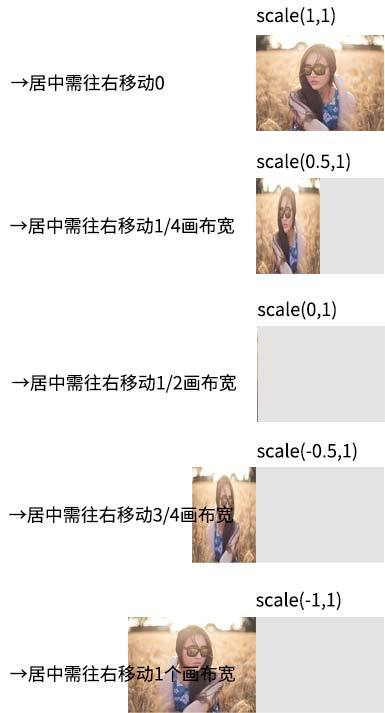## 在canvas上实现元素图片镜像翻转动画效果的方法，

demo页面中点击图片动画效果可见。

CSS中要想实现元素的翻转效果，比较简单，例如我们希望某一张图片水平镜像翻转，只需要一行CSS就可以了：

```img {
transform: scaleX(-1);
}```

```img {
transform: scale(-1, 1);
}```

`context.scale(-1, 1);`

```context.setTransform(-1, 0, 0, 1, 0, 0);
```

canvas默认的变化坐标系是左上角。`distance = (canvas.width – image.width * scale) / 2;`

```// 坐标参考调整
context.translate((canvas.width - image.width * scale) / 2, 0);
context.scale(scale, 1);
context.drawImage(image, 0, 0);
// 坐标参考还原
context.setTransform(1, 0, 0, 1, 0, 0);```

`Math.animation(form, to, duration, easing, callback);`

```var canvas = document.querySelector('canvas');
var context = canvas.getContext('2d');
// 动画进行
Math.animation(1, -1, 600, 'Quad.easeInOut', function (value, isEnding) {
// 清除画布内容
context.clearRect(0, 0, canvas.width, canvas.height);
// 调整坐标
context.translate((canvas.width - canvas.width * value) / 2, 0);
// 调整缩放
context.scale(value, 1);
// 绘制此时图片
context.drawImage(eleImg, 0, 0);
// 坐标参考还原
context.setTransform(1, 0, 0, 1, 0, 0);
});
```

暂无相关文章